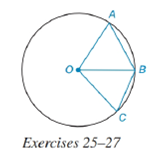Chapter 6.4, Problem 25E### Elementary Geometry for College St...

6th Edition
Daniel C. Alexander + 1 other
ISBN: 9781285195698

#### Solutions

Chapter
Section### Elementary Geometry for College St...

6th Edition
Daniel C. Alexander + 1 other
ISBN: 9781285195698
Textbook Problem
1 views

#In ⊙ O , m ∠ A O B = 70 ° and m ∠ B O C = 30 ° . See the figure above.a) How are m A B ⌢ and m B C ⌢ related?b) How are A B and B C related?

To determine

(a)

To find:

The relation between mAB and mBC.

Explanation

Given:

O, mAOB=70° and mBOC=30°. The figure given below

Theorem Used:

According to the following theorem

In a circle (or in congruent circles) containing two unequal central angles, the larger angle corresponds to the larger intercepted arc.

Calculation:

Using the theorem:

In a circle (or in congruent circles) containing two unequal central angles, the larger angle corresponds to the larger intercepted arc.

Since the angles mAOB=70° and mBOC=30° are unequal and mBOC<mAOB and from the given figure,

To determine

(b)

To find:

The relation between AB and BC.

### Still sussing out bartleby?

Check out a sample textbook solution.

See a sample solution

#### The Solution to Your Study Problems

Bartleby provides explanations to thousands of textbook problems written by our experts, many with advanced degrees!

Get Started

#### Evaluate the expression sin Exercises 116. 52535252

Finite Mathematics and Applied Calculus (MindTap Course List)

#### Subtract: 2301506

Elementary Technical Mathematics

#### True or False: The figure at the right is the graph of the direction field for y = (y 1)x2.

Study Guide for Stewart's Single Variable Calculus: Early Transcendentals, 8th

#### True or False: , for –π ≤ t ≤ π, is smooth.

Study Guide for Stewart's Multivariable Calculus, 8th